##### SAT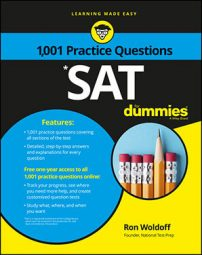Chances are good that the SAT Math exam will ask you to solve a problem involving partial areas. In these questions, you're generally given a shaded area within another area, and asked to calculate just the shaded area.

The following practice questions ask you to calculate shaded areas involving circles, squares, and triangles. Be careful, though: one of the questions may be a trap.

## Practice questions

1. A square is inscribed within a circle.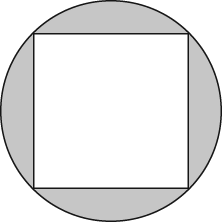If it has a side length of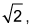what is the area of the shaded portion of the drawing?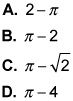2. What is the area of the shaded triangle?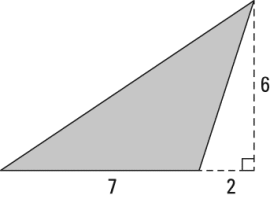A. 54 B. 42 C. 27 D. 21

1. The correct answer is Choice (B). First find the area of the square: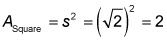For the area of the circle, you need its radius. Cut the square in half, corner to corner, to form two 45-45-90 triangles, where each hypotenuse is the diameter of the circle. If the side of this triangle is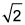the hypotenuse is 2, because in a right triangle, the square of the hypotenuse is the sum of the squares of the other two sides: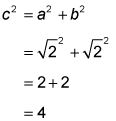c2 = 4, so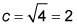is the diameter of the circle, and the radius of the circle is half the diameter, or 1. Now for the area of the circle: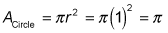Subtract the area of the square from the area of the circle for your answer: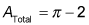2. The correct answer is Choice (D). For the area of a triangle, multiply the base by the height and divide by 2. The base of this triangle is 7, and the height is 6, for an area of 21. The 2 in the drawing has no bearing.